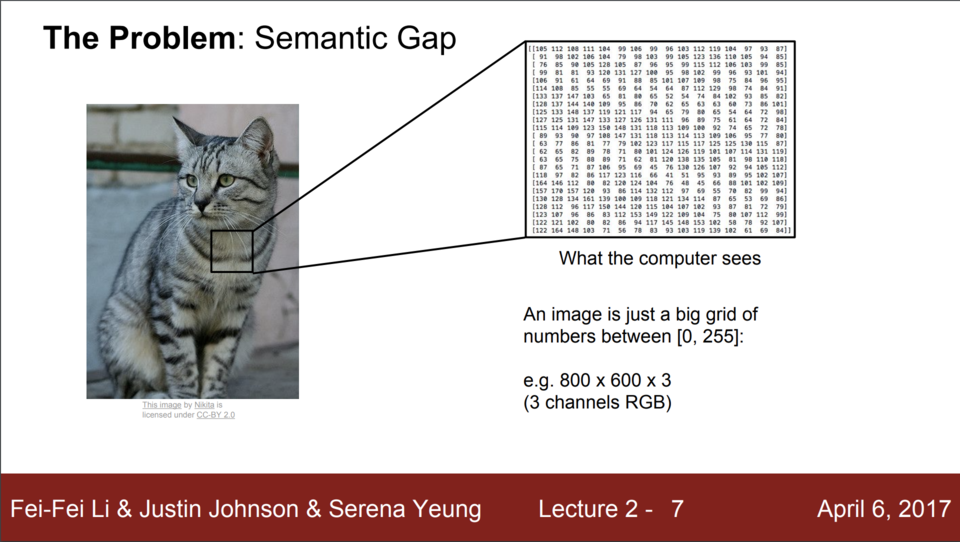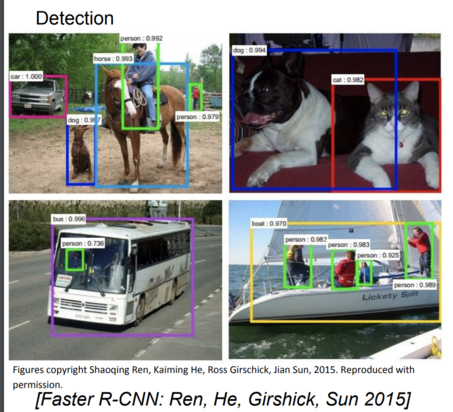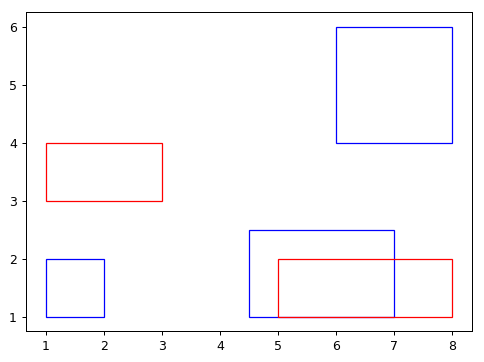# 用矩阵操作快速实现图像识别中 Bounding Box 的准确率和召回率判断

## 背景

### 像素坐标系### Bounding Box## 问题

### 问题的提出


import numpy as np

# create array data

predict = np.array([[1,2,2,1],
[4.5,2.5,2,1],
[6,6,8,4]], np.double)

truth = np.array([[1,4,3,3],
[5,2,8,1]], np.double)

# Below is to show the layout of the problem
# red represents truth
# blue represents prediction

import matplotlib.pyplot as plt
import matplotlib.patches as patches
fig = plt.figure()
recList = list()

# Adding blue rectangle from predict
for rect in predict:
recList.append(
patches.Rectangle(
(rect, rect),
np.abs(rect - rect),
np.abs(rect - rect),
fill=False,
edgecolor = "blue"
)
)

# Adding red rectangle for truth
for rect in truth:
recList.append(
patches.Rectangle(
(rect, rect),
np.abs(rect - rect),
np.abs(rect - rect),
fill=False,
edgecolor = "red"
)
)

# plot the graph
for p in recList:

plt.plot()
plt.show()
fig.savefig('rect.png', dpi=90, bbox_inches='tight')'''
Calculate the center point of bounding box
'''
def bbox2centroid(bboxes):
return np.column_stack(((bboxes[:, 0] + bboxes[:, 2])/2, (bboxes[:, 1] + bboxes[:, 3])/2))



### 问题的思路

#### numpy 的 numpy.newaxis

numpyNone 也可以作为矩阵一个新的维度的占位符。用官方文档的说法，这叫做 numpy.newaxis

The newaxis object can be used in all slicing operations to create an axis of length one. :const: newaxis is an alias for ‘None’, and ‘None’ can be used in place of this with the same result.

A=np.array([1,3,5])

print(A)
print(A.shape)

print("====1====")

print(A[:,None])
print(A[:,None].shape)

print("====2====")

print(A[None,:])
print(A[None,:].shape)



[1 3 5]
(3,)
====1====
[

]
(3, 1)
====2====
[[1 3 5]]
(1, 3)



A=np.array([[1,3,5],
[7,8,9]])

print(A)
print(A.shape)

print("====1====")

print(A[:,None])
print(A[:,None].shape)

print("====2====")

print(A[None,:])
print(A[None,:].shape)


[[1 3 5]
[7 8 9]]
(2, 3)
====1====
[[[1 3 5]]

[[7 8 9]]]
(2, 1, 3)
====2====
[[[1 3 5]
[7 8 9]]]
(1, 2, 3)



### 问题的解决代码


'''
Calculating P and R function
'''
def compute_score_detail_by_centroid(pred_bbox, gt_bbox, tolerence=(2, 2)):
pred_c = bbox2centroid(pred_bbox)
gt_c = bbox2centroid(gt_bbox)
diffs = abs(pred_c[:, None] - gt_c)
x1, x2 = np.nonzero((diffs < tolerence).all(2))
precision = np.unique(x1).shape / pred_bbox.shape
recall = np.unique(x2).shape / gt_bbox.shape
return precision, recall


(1) pred_c = bbox2centroid(pred_bbox) 和 gt_c = bbox2centroid(gt_bbox) 就是求 Bounding Box 的中心，在我们的例子中，做完这步操作， pred_c 是 $$3 \times 2$$ 矩阵 而 gt_c 是 $$2 \times 2$$ 矩阵。

[[1.5  1.5 ]
[3.25 1.75]
[7.   5.  ]]


gt_c

[[2.  3.5]
[6.5 1.5]]


(2) pred_c[:, None] 将 $$3 \times 2$$ 矩阵 扩展为 $$3 \times 1 \times 2$$ 矩阵

(3) pred_c[:, None] - gt_c 是 $$3 \times 1 \times 2$$ 矩阵 减去 $$2 \times 2$$ 矩阵， 在数学上这是没有定义的，因为数学要求矩阵减法操作必须维度相同，但是在 numpy 中，其实是把 $$3 \times 1 \times 2$$ 矩阵作为 3 个 $$1 \times 2$$ 矩阵分别先扩充为 $$2 \times 2$$ 矩阵 再和$$2 \times 2$$ 的 gt_c 矩阵做减法。


[[[0.5  2.  ]
[5.   0.  ]]

[[1.25 1.75]
[3.25 0.25]]

[[5.   1.5 ]
[0.5  3.5 ]]]




'''
Calculating P and R function
'''
def compute_score_detail_by_centroid(pred_bbox, gt_bbox, tolerence=(2, 2)):
pred_c = bbox2centroid(pred_bbox)
print(pred_c)
gt_c = bbox2centroid(gt_bbox)
print(gt_c)
diffs = abs(pred_c[:, None] - gt_c)
print(diffs)
print(diffs.shape)
x1, x2 = np.nonzero((diffs < tolerence).all(2))
print((diffs < tolerence).all(2))
print(np.nonzero((diffs < tolerence).all(2)))
precision = np.unique(x1).shape / pred_bbox.shape
recall = np.unique(x2).shape / gt_bbox.shape
return precision, recall



(4) (diffs < tolerence).all(2) 这步就是把上面求出来的 $$3 \times 2 \times 2$$ 矩阵 和 我们允许的误差矩阵((2, 2))做比较，如果小于误差计作True，否则计作 False。但是这样结束之后我们得到一个$$3 \times 2 \times 2$$ 的布尔(Boolean)矩阵。

[[[ True False]
[False  True]]

[[ True  True]
[False  True]]

[[False  True]
[ True False]]]




[[False False]
[ True False]
[False False]](4) x1, x2 = np.nonzero((diffs < tolerence).all(2)) 主要需要理解np.nonzero(),其实就是返回每一个维度的非零元素，在我们这个布尔矩阵中就是返回每个维度的True数目。Return the indices of the elements that are non-zero.Returns a tuple of arrays, one for each dimension of a, containing the indices of the non-zero elements in that dimension.

$$N \times 2$$ 的预测矩阵和 $$M \times 2$$ 真实值矩阵的差是不是在允许的范围内。

## 总结

Written on April 30, 2018